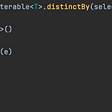# Ideas and Solutions for Advent of Code 2021 in Kotlin — Part 4/4

The final week of Advent of Code was, obviously, the most challenging one. To be honest I was close to being happy with just a silver star multiple times. But at the end, tenacity made the difference. 50 stars are mine this year 🌟

Ideas and Solutions for tasks 1 to 6 can be found here, for tasks 7 to 12 — here, for tasks 13 to 18 — here.

## Day 19: Beacon Scanner

We are provided with several sets of points in 3D space. Those points are rotated differently in each set, so we don't know how X, Y, and Z coordinates are aligned between sets. Our goal is to merge all those sets into a single group by matching points (sub-task one) and find the most significant distance between coordinate origins after they are matched. Here is the complete task.

This is an exciting task! I've initially learned about this idea from The Three-Body Problem book, which introduced the concept of locating any star in the Universe by the distances from a few nearest stars — in our case, "a few" means 12. Think about it as a star fingerprint ;)

So to match the sets of points in 3D space, we need:

• check all combinations of rotations in three dimensions (in our task, the step is 90 degrees, so there are 48 combinations) for the whole set
• assume that each pair of points from the first set and the second set is an intersection by adjusting all points to that pair
• check how many adjusted points intersect between the two sets
• if there are more than 12 intersections, voilá — the sets are matched

The embedded Kotlin's functions like `intersect`, `map`, and `mapNotNull` make the solution, even for such a complex task, elegant.

## Day 20: Trench Map

This image-enhancing task provides us with the initial photo (2D array of black and white pixels), and enhancing guidance (1D array). Enhancing happens in steps, where for each pixel, we found its enhanced value as a result of a combination of itself + all eight adjacent pixels. Here is the complete task.

We go from the left to right and top to bottom to solve this task and enhance each pixel. The first complication is that input is considered infinite, so we are unsure how many pixels to enhance. But if you think about it, you quickly notice that the actual picture grows only one pixel per time. So adding padding equal to a number of steps on all sides of the 2D array solves the problem.

The second complication is that a combination of nine white pixels or nine black pixels may blink (change the value to the opposite), making the whole infinite input blink. This problem is solved by assuming all endless input blinks the same way as padding. So we just use the top-left value of padding to replace the infinite input when needed.

Here is my solution.

## Day 21: Dirac Dice

Two players throw three dice in turn and then move their pawn by the total of those three dice values. Pawns move on the circular field with ten possible positions (1 to 10). There are initial positions for each player's pawn. Each position at the end of each turn adds to the player's score. The first player who reaches the target score wins. Here is the complete task.

In the first sub-task, dice values always go from 1 to 100, which is extremely predictable and easily modeled. We need to calculate how many times dice will be thrown before one of the players wins and multiply it by the lower score.

In the second sub-task, it's a real dice with values from 1 to 3, which are randomly drawn. We need to calculate how many unique games will the winning player win. A unique game means the difference in at least one dice value.

This means there are 27 (3³) variants per one player turn or 729 (3⁶) variants per one game turn (two players throw six dices). So assuming there are max ten game turns to reach the score of 21, we get 3⁶⁰ variants of the game. Not way the modeling can solve that.

But the dynamic programming can 😉 Consider 5D array describing the following state `d[step][position1][position2][score1][score2]`. We know the initial positions of both players as well as their scores. Let it be the initial state — `d[firstPosition][secondPosition] = 1`. Yes, there is just one variant to start with.

Now we will be moving from step 1 to step 10. For every position on which player one and player two has their scores, we roll the first dice triple to see if it reaches the target score. If so, we update the corresponding value of the current step with updated position and score for the first player by multiplying the value from the previous step by the probability of this roll.

Otherwise, we roll the second dice triple and reassign the corresponding value of the current step with updated position and score for the first and second player by multiplying the value from the previous step by the combined (multiplied) probability of both roles.

After that, we just need to sum up all the values where the score of player one is bigger or equal than the target value, and the score of player two where the score of player one is less than the target value. Not much Kotlin in this one.

Here is my solution.

## Day 22: Reactor Reboot

We are provided with a set of "on" and "off" cube operations, which are handled sequentially and may intersect. After all operations are executed, our goal is to calculate how many "on" cubes of size 1x1x1 we will have. Here is the complete task.

The first sub-task can be modeled on the real 3D array. But in the second sub-task, coordinates are too large, so we need to find a more optimal solution.

Such a solution can be based on the following approach:

• keep the list of all big "on" and "off" cubes
• for each operation, add intersections with cubes from that list to the list with an inverse type
• if it's an "on" operation, add it as a cube to the list as well
• in the end, just calculate the sum of all cubes in the list

Here is my solution.

## Day 23: Amphipod

There are four types of amphipods (A, B, C, D) located in 4 random rooms at the start. We should move them to the target rooms (A -> room 1, B -> room 2, C -> room 3, D -> room 4) using the hall as a buffer. There are specific rules that are better to discover reading the complete task.

It seems to be an NP-complete task, which means that you should check all the possible moves to find the best solution. A recursive solution with an A* search algorithm or caching should work.

But I (as many other people if we look on Reddit) couldn't stand trying to find the best solution myself because it seems so much like a puzzle. So yeah, this time, no code on my side, but here is my solution, anyway.

## Day 24: Arithmetic Logic Unit

We get a set of mathematical instructions on four variables which we need to execute on different 14-digit numbers. As a result, we need to calculate the minimum and maximum 14-digit numbers, which satisfy the condition (the end value of one variable should be zero). Here is the complete task.

By looking at the set of instructions to execute, it's easy to see that they can be split into 14 sequences, mostly the same. The only difference is about `div z`, `add x`, and `add y` operations that have different constants.

Also, it's easy to see that the previous `z` and the new digit are used as input values for the next step. It hints about the reverse dynamic programming solution, where we move from the end state of `z` (zero) to the start state to see which `z` values are possible for each digit.

Then by having those transitions, we can generate all 14-digit numbers that fit the condition and choose min and max values from that list.

Here is my solution.

## Day 25: Sea Cucumber

The Last Day of the Advent! So rather the simple task — model the cycle movements of two different types, from top to bottom and left to right, on the 2D array. Here is the complete task.

The only complexity we need to overcome is that movements always happen in two phases — from left to right, and only then from top to bottom taking into account freed and taken cells from the previous phase. Instead of the second sub-task, you need … 49 stars, which means solving all previous tasks.

Here is my solution.

## Bottom line

That’s it for this year’s Advent of Code, see you next year! I find it a great opportunity to practice problem-solving skills, learn new data structures and algorithms, and just have fun.

It’s exciting to see that all the tasks can be solved in Kotlin, the language we are using every day on our work for mobile, backend, and frontend development. Great work JetBrains Team Blog!

--

--

--

## More from xorum.io

Kotlin Multiplatform solutions for Mobile (iOS | Android) and Web (Ktor | React.js)

## Measuring Web Performance Tools 101## Add files to Azure DevOps Git repository using C# (REST API call)## High Availability Architecture With AWS CLI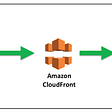## From ‘lines of code’ to ‘the bottom line’; outsourcing companies that add value## Koin Android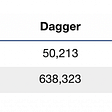## CS373 Spring 2022: Christopher Carrasco — Week 6## Crust Network and Kaka Collaborate For NFT Asset Decentralized Storage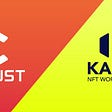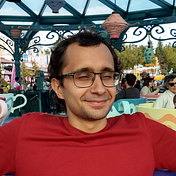## Yev Kanivets

Professional Mobile Engineer since 2014. Kotlin Multiplatform enthusiast. Love hackathons, competitive programming, and challenging tasks. Marathon finisher.

## Analyzing the algorithmic complexity of the Kotlin API’s distinctBy function